# NCERT Class 8 Maths Chapter 14 – Factorisation solutions

## Exercise 14.1 Page No: 208

1. Find the common factors of the given terms.

(i) 12x, 36

(ii) 2y, 22xy

(iii) 14 pq, 28p2q2

(iv) 2x, 3x2, 4

(v) 6 abc, 24ab2, 12a2b

(vi) 16 x3, – 4x2 , 32 x

(vii) 10 pq, 20qr, 30 rp

(viii) 3x2y3 , 10x3y2 , 6x2y2z

Solution:

(i) Factors of 12x and 36

12x = 2×2×3×x

36 = 2×2×3×3

Common factors of 12x and 36 are 2, 2, 3

and , 2×2×3 = 12

(ii) Factors of 2y and 22xy

2y = 2×y

22xy = 2×11×x×y

Common factors of 2y and 22xy are 2, y

and ,2×y = 2y

(iii) Factors of 14pq and 28p2q

14pq = 2x7xpxq

28p2q = 2x2x7xpxpxq

Common factors of 14 pq and 28 p2q are 2, 7 , p , q

and, 2x7xpxq = 14pq

(iv) Factors of 2x, 3x2and 4

2x = 2×x

3x2= 3×x×x

4 = 2×2

Common factors of 2x, 3xand 4 is 1.

(v) Factors of 6abc, 24ab2 and 12a2b

6abc = 2×3×a×b×c

24ab2 = 2×2×2×3×a×b×b

12 ab = 2×2×3×a×a×b

Common factors of 6 abc, 24ab2 and 12a2b are 2, 3, a, b

and, 2×3×a×b = 6ab

(vi) Factors of 16x, -4x2and 32x

16 x= 2×2×2×2×x×x×x

– 4x2 = -1×2×2×x×x

32x = 2×2×2×2×2×x

Common factors of 16 x, – 4xand 32x are 2,2, x

and, 2×2×x = 4x

(vii) Factors of 10 pq, 20qr and 30rp

10 pq = 2×5×p×q

20qr = 2×2×5×q×r

30rp= 2×3×5×r×p

Common factors of 10 pq, 20qr and 30rp are 2, 5

and, 2×5 = 10

(viii) Factors of 3x2y3 , 10x3y2 and 6x2y2z

3x2y3 = 3×x×x×y×y×y

10xy2 = 2×5×x×x×x×y×y

6x2y2z = 3×2×x×x×y×y×z

Common factors of 3x2y3, 10x3y2 and 6x2y2z are x2, y2

and, x2×y2 = x2y2

2.Factorise the following expressions

(i) 7x–42

(ii) 6p–12q

(iii) 7a2+ 14a

(iv) -16z+20 z3

(v) 20l2m+30alm

(vi) 5x2y-15xy2

(vii) 10a2-15b2+20c2

(viii) -4a2+4ab–4 ca

(ix) x2yz+xy2z +xyz2

(x) ax2y+bxy2+cxyz

Solution: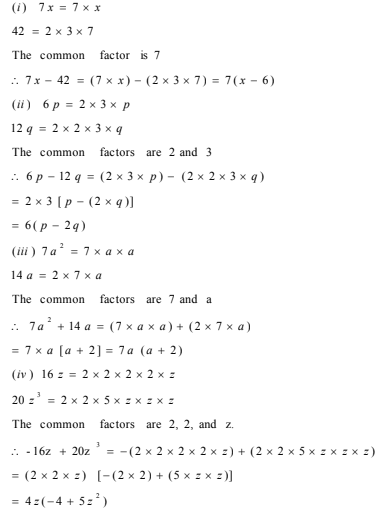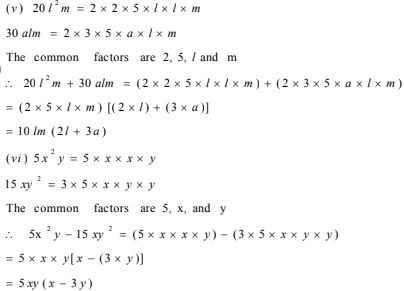(vii) 10a2-15b2+20c2

10a= 2×5×a×a

– 15b= -1×3×5×b×b

20c2 = 2×2×5×c×c

Common factor of 10 a2 , 15b2 and 20c2 is 5

10a2-15b2+20c2 = 5(2a2-3b2+4c2 )

(viii) – 4a2+4ab-4ca

– 4a2 = -1×2×2×a×a

4ab = 2×2×a×b

– 4ca = -1×2×2×c×a

Common factor of – 4a2 , 4ab , – 4ca are 2, 2, a i.e. 4a

So,

– 4a2+4 ab-4 ca = 4a(-a+b-c)

(ix) x2yz+xy2z+xyz2

x2yz = x×x×y×z

xy2z = x×y×y×z

xyz2 = x×y×z×z

Common factor of x2yz , xy2z and xyz2 are x, y, z i.e. xyz

Now, x2yz+xy2z+xyz= xyz(x+y+z)

(x) ax2y+bxy2+cxyz

ax2y = a×x×x×y

bxy= b×x×y×y

cxyz = c×x×y×z

Common factors of a x2y ,bxyand cxyz are xy

Now, ax2y+bxy2+cxyz = xy(ax+by+cz)

3. Factorise.

(i) x2+xy+8x+8y

(ii) 15xy–6x+5y–2

(iii) ax+bx–ay–by

(iv) 15pq+15+9q+25p

(v) z–7+7xy–xyz

Solution: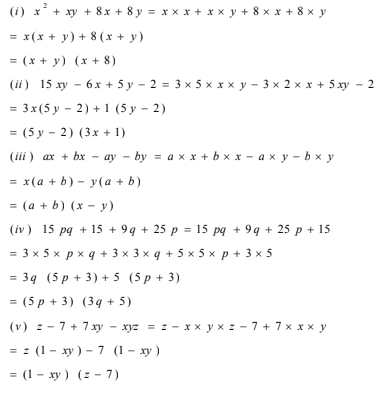## Exercise 14.2 Page No: 223

1. Factorise the following expressions.

(i) a2+8a+16

(ii) p2–10p+25

(iii) 25m2+30m+9

(iv) 49y2+84yz+36z2

(v) 4x2–8x+4

(vi) 121b2–88bc+16c2

(vii) (l+m)2–4lm (Hint: Expand (l+m)2 first)

(viii) a4+2a2b2+b4

Solution:

(i) a2+8a+16

= a2+2×4×a+42

= (a+4)2

Using identity: (x+y)2 = x2+2xy+y2

(ii) p2–10p+25

= p2-2×5×p+52

= (p-5)2

Using identity: (x-y)2 = x2-2xy+y2

(iii) 25m2+30m+9

= (5m)2-2×5m×3+32

= (5m+3)2

Using identity: (x+y)2 = x2+2xy+y2

(iv) 49y2+84yz+36z2

=(7y)2+2×7y×6z+(6z)2

= (7y+6z)2

Using identity: (x+y)2 = x2+2xy+y2

(v) 4x2–8x+4

= (2x)2-2×4x+22

= (2x-2)2

Using identity: (x-y)2 = x2-2xy+y2

(vi) 121b2-88bc+16c2

= (11b)2-2×11b×4c+(4c)2

= (11b-4c)2

Using identity: (x-y)2 = x2-2xy+y2

(vii) (l+m)2-4lm (Hint: Expand (l+m)2 first)

Expand (l+m)using identity: (x+y)2 = x2+2xy+y2

(l+m)2-4lm = l2+m2+2lm-4lm

= l2+m2-2lm

= (l-m)2

Using identity: (x-y)2 = x2-2xy+y2

(viii) a4+2a2b2+b4

= (a2)2+2×ab2+(b2)2

= (a2+b2)2

Using identity: (x+y)2 = x2+2xy+y2

2. Factorise.

(i) 4p2–9q2

(ii) 63a2–112b2

(iii) 49x2–36

(iv) 16x5–144x3 differ

(v) (l+m)2-(l-m) 2

(vi) 9x2y2–16

(vii) (x2–2xy+y2)–z2

(viii) 25a2–4b2+28bc–49c2

Solution:

(i) 4p2–9q2

= (2p)2-(3q)2

= (2p-3q)(2p+3q)

Using identity: x2-y2 = (x+y)(x-y)

(ii) 63a2–112b2

= 7(9a2 –16b2)

= 7((3a)2–(4b)2)

= 7(3a+4b)(3a-4b)

Using identity: x2-y2 = (x+y)(x-y)

(iii) 49x2–36

= (7a)2 -62

= (7a+6)(7a–6)

Using identity: x2-y2 = (x+y)(x-y)

(iv) 16x5–144x3

= 16x3(x2–9)

= 16x3(x2–9)

= 16x3(x–3)(x+3)

Using identity: x2-y2 = (x+y)(x-y)

(v) (l+m) 2-(l-m) 2

= {(l+m)-(l–m)}{(l +m)+(l–m)}

Using Identity: x2-y2 = (x+y)(x-y)

= (l+m–l+m)(l+m+l–m)

= (2m)(2l)

= 4 ml

(vi) 9x2y2–16

= (3xy)2-42

= (3xy–4)(3xy+4)

Using Identity: x2-y2 = (x+y)(x-y)

(vii) (x2–2xy+y2)–z2

= (x–y)2–z2

Using Identity: (x-y)2 = x2-2xy+y2

= {(x–y)–z}{(x–y)+z}

= (x–y–z)(x–y+z)

Using Identity: x2-y2 = (x+y)(x-y)

(viii) 25a2–4b2+28bc–49c2

= 25a2–(4b2-28bc+49c2 )

= (5a)2-{(2b)2-2(2b)(7c)+(7c)2}

= (5a)2-(2b-7c)2

Using Identity: x2-y2 = (x+y)(x-y) , we have

= (5a+2b-7c)(5a-2b-7c)

3. Factorise the expressions.

(i) ax2+bx

(ii) 7p2+21q2

(iii) 2x3+2xy2+2xz2

(iv) am2+bm2+bn2+an2

(v) (lm+l)+m+1

(vi) y(y+z)+9(y+z)

(vii) 5y2–20y–8z+2yz

(viii) 10ab+4a+5b+2

(ix)6xy–4y+6–9x

Solution:

(i) ax2+bx = x(ax+b)

(ii) 7p2+21q2 = 7(p2+3q2)

(iii) 2x3+2xy2+2xz2 = 2x(x2+y2+z2)

(iv) am2+bm2+bn2+an= m2(a+b)+n2(a+b) = (a+b)(m2+n2)

(v) (lm+l)+m+1 = lm+m+l+1 = m(l+1)+(l+1) = (m+1)(l+1)

(vi) y(y+z)+9(y+z) = (y+9)(y+z)

(vii) 5y2–20y–8z+2yz = 5y(y–4)+2z(y–4) = (y–4)(5y+2z)

(viii) 10ab+4a+5b+2 = 5b(2a+1)+2(2a+1) = (2a+1)(5b+2)

(ix) 6xy–4y+6–9x = 6xy–9x–4y+6 = 3x(2y–3)–2(2y–3) = (2y–3)(3x–2)

4.Factorise.

(i) a4–b4

(ii) p4–81

(iii) x4–(y+z) 4

(iv) x4–(x–z) 4

(v) a4–2a2b2+b4

Solution:

(i) a4–b4

= (a2)2-(b2)2

= (a2-b2) (a2+b2)

= (a – b)(a + b)(a2+b2)

(ii) p4–81

= (p2)2-(9)2

= (p2-9)(p2+9)

= (p2-32)(p2+9)

=(p-3)(p+3)(p2+9)

(iii) x4–(y+z) 4 = (x2)2-[(y+z)2]2

= {x2-(y+z)2}{ x2+(y+z)2}

= {(x –(y+z)(x+(y+z)}{x2+(y+z)2}

= (x–y–z)(x+y+z) {x2+(y+z)2}

(iv) x4–(x–z) 4 = (x2)2-{(x-z)2}2

= {x2-(x-z)2}{x2+(x-z)2}

= { x-(x-z)}{x+(x-z)} {x2+(x-z)2}

= z(2x-z)( x2+x2-2xz+z2)

= z(2x-z)( 2x2-2xz+z2)

(v) a4–2a2b2+b4 = (a2)2-2a2b2+(b2)2

= (a2-b2)2

= ((a–b)(a+b))2

5. Factorise the following expressions.

(i) p2+6p+8

(ii) q2–10q+21

(iii) p2+6p–16

Solution:

(i) p2+6p+8

We observed that, 8 = 4×2 and 4+2 = 6

p2+6p+8 can be written as p2+2p+4p+8

Taking Common terms, we get

p2+6p+8 = p2+2p+4p+8 = p(p+2)+4(p+2)

Again p+2 is common in both the terms.

= (p+2)(p+4)

This implies: p2+6p+8 = (p+2)(p+4)

(ii) q2–10q+21

Observed that, 21 = -7×-3 and -7+(-3) = -10

q2–10q+21 = q2–3q-7q+21

= q(q–3)–7(q–3)

= (q–7)(q–3)

This implies q2–10q+21 = (q–7)(q–3)

(iii) p2+6p–16

We observed that, -16 = -2×8 and 8+(-2) = 6

p2+6p–16 = p2–2p+8p–16

= p(p–2)+8(p–2)

= (p+8)(p–2)

So, p2+6p–16 = (p+8)(p–2)

## Exercise 14.3 Page No: 227

1. Carry out the following divisions.

(i) 28x4 ÷ 56x

(ii) –36y3 ÷ 9y2

(iii) 66pq2r3 ÷ 11qr2

(iv) 34x3y3z3 ÷ 51xy2z3

(v) 12a8b8 ÷ (– 6a6b4)

Solution:

(i)28x4 = 2×2×7×x×x×x×x

56x = 2×2×2×7×x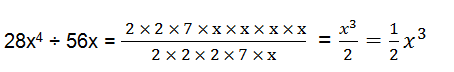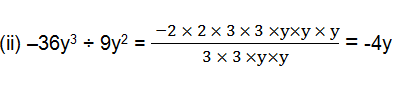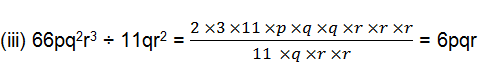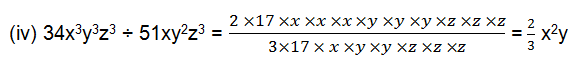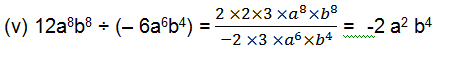2. Divide the given polynomial by the given monomial.

(i)(5x2–6x) ÷ 3x

(ii)(3y8–4y6+5y4) ÷ y4

(iii) 8(x3y2z2+x2y3z2+x2y2z3)÷ 4xyz2

(iv)(x3+2x2+3x) ÷2x

(v) (p3q6–p6q3) ÷ p3q3

Solution: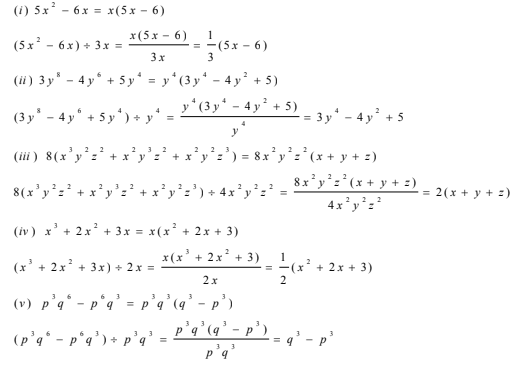3. Work out the following divisions.

(i) (10x–25) ÷ 5

(ii) (10x–25) ÷ (2x–5)

(iii) 10y(6y+21) ÷ 5(2y+7)

(iv) 9x2y2(3z–24) ÷ 27xy(z–8)

(v) 96abc(3a–12)(5b–30) ÷ 144(a–4)(b–6)

Solution:

(i) (10x–25) ÷ 5 = 5(2x-5)/5 = 2x-5

(ii) (10x–25) ÷ (2x–5) = 5(2x-5)/( 2x-5) = 5

(iii) 10y(6y+21) ÷ 5(2y+7) = 10y×3(2y+7)/5(2y+7) = 6y

(iv) 9x2y2(3z–24) ÷ 27xy(z–8) = 9x2y2×3(z-8)/27xy(z-8) = xy4. Divide as directed.

(i) 5(2x+1)(3x+5)÷ (2x+1)

(ii) 26xy(x+5)(y–4)÷13x(y–4)

(iii) 52pqr(p+q)(q+r)(r+p) ÷ 104pq(q+r)(r+p)

(iv) 20(y+4) (y2+5y+3) ÷ 5(y+4)

(v) x(x+1) (x+2)(x+3) ÷ x(x+1)

Solution: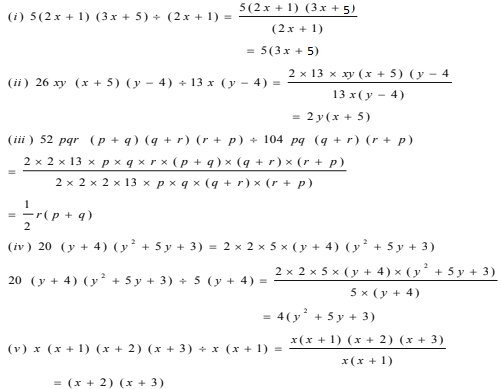5. Factorise the expressions and divide them as directed.

(i) (y2+7y+10)÷(y+5)

(ii) (m2–14m–32)÷(m+2)

(iii) (5p2–25p+20)÷(p–1)

(iv) 4yz(z2+6z–16)÷2y(z+8)

(v) 5pq(p2–q2)÷2p(p+q)

(vi) 12xy(9x2–16y2)÷4xy(3x+4y)

(vii) 39y3(50y2–98) ÷ 26y2(5y+7)

Solution:

(i) (y2+7y+10)÷(y+5)

First solve for equation, (y2+7y+10)

(y2+7y+10) = y2+2y+5y+10 = y(y+2)+5(y+2) = (y+2)(y+5)

Now, (y2+7y+10)÷(y+5) = (y+2)(y+5)/(y+5) = y+2

(ii) (m2–14m–32)÷ (m+2)

Solve for m2–14m–32, we have

m2–14m–32 = m2+2m-16m–32 = m(m+2)–16(m+2) = (m–16)(m+2)

Now, (m2–14m–32)÷(m+2) = (m–16)(m+2)/(m+2) = m-16

(iii) (5p2–25p+20)÷(p–1)

Step 1: Take 5 common from the equation, 5p2–25p+20, we get

5p2–25p+20 = 5(p2–5p+4)

Step 2: Factorize p2–5p+4

p2–5p+4 = p2–p-4p+4 = (p–1)(p–4)

Step 3: Solve original equation

(5p2–25p+20)÷(p–1) = 5(p–1)(p–4)/(p-1) = 5(p–4)

(iv) 4yz(z2 + 6z–16)÷ 2y(z+8)

Factorize z2+6z–16,

z2+6z–16 = z2-2z+8z–16 = (z–2)(z+8)

Now, 4yz(z2+6z–16) ÷ 2y(z+8) = 4yz(z–2)(z+8)/2y(z+8) = 2z(z-2)

(v) 5pq(p2–q2) ÷ 2p(p+q)

p2–q2 can be written as (p–q)(p+q) using identity.

5pq(p2–q2) ÷ 2p(p+q) = 5pq(p–q)(p+q)/2p(p+q) = 5/2q(p–q)

(vi) 12xy(9x2–16y2) ÷ 4xy(3x+4y)

Factorize 9x2–16y2 , we have

9x2–16y2 = (3x)2–(4y)2 = (3x+4y)(3x-4y) using identity: p2–q2 = (p–q)(p+q)

Now, 12xy(9x2–16y2) ÷ 4xy(3x+4y) = 12xy(3x+4y)(3x-4y) /4xy(3x+4y) = 3(3x-4y)

(vii) 39y3(50y2–98) ÷ 26y2(5y+7)
st solve for 50y2–98, we have

50y2–98 = 2(25y2–49) = 2((5y)2–72) = 2(5y–7)(5y+7)

Now, 39y3(50y2–98) ÷ 26y2(5y+7) =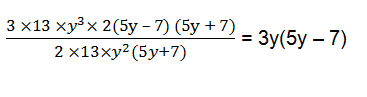## Exercise 14.4 Page No: 228

1. 4(x–5) = 4x–5

Solution:

4(x- 5)= 4x – 20 ≠ 4x – 5 = RHS

The correct statement is 4(x-5) = 4x–20

2. x(3x+2) = 3x2+2

Solution:

LHS = x(3x+2) = 3x2+2x ≠ 3x2+2 = RHS

The correct solution is x(3x+2) = 3x2+2x

3. 2x+3y = 5xy

Solution:

LHS= 2x+3y ≠ R. H. S

The correct statement is 2x+3y = 2x+3 y

4. x+2x+3x = 5x

Solution:

LHS = x+2x+3x = 6x ≠ RHS

The correct statement is x+2x+3x = 6x

5. 5y+2y+y–7y = 0

Solution:

LHS = 5y+2y+y–7y = y ≠ RHS

The correct statement is 5y+2y+y–7y = y

6. 3x+2x = 5x2

Solution:

LHS = 3x+2x = 5x ≠ RHS

The correct statement is 3x+2x = 5x

7. (2x) 2+4(2x)+7 = 2x2+8x+7

Solution:

LHS = (2x) 2+4(2x)+7 = 4x2+8x+7 ≠ RHS

The correct statement is (2x) 2+4(2x)+7 = 4x2+8x+7

8. (2x) 2+5x = 4x+5x = 9x

Solution:

LHS = (2x) 2+5x = 4x2+5x ≠ 9x = RHS

The correct statement is(2x) 2+5x = 4x2+5x

9. (3x + 2) 2 = 3x2+6x+4

Solution:

LHS = (3x+2) 2 = (3x)2+22+2x2x3x = 9x2+4+12x ≠ RHS

The correct statement is (3x + 2) 2 = 9x2+4+12x

10. Substituting x = – 3 in

(a) x2 + 5x + 4 gives (– 3) 2+5(– 3)+4 = 9+2+4 = 15

(b) x2 – 5x + 4 gives (– 3) 2– 5( – 3)+4 = 9–15+4 = – 2

(c) x2 + 5x gives (– 3) 2+5(–3) = – 9–15 = – 24

Solution:

(a) Substituting x = – 3 in x2+5x+4, we have

x2+5x+4 = (– 3) 2+5(– 3)+4 = 9–15+4 = – 2. This is the correct answer.

(b) Substituting x = – 3 in x2–5x+4

x2–5x+4 = (–3) 2–5(– 3)+4 = 9+15+4 = 28. This is the correct answer

(c)Substituting x = – 3 in x2+5x

x2+5x = (– 3) 2+5(–3) = 9–15 = -6. This is the correct answer

11.(y–3)2 = y2–9

Solution:

LHS = (y–3)2 , which is similar to (a–b)2 identity, where (a–b) 2 = a2+b2-2ab.

(y – 3)2 = y2+(3) 2–2y×3 = y2+9 –6y ≠ y2 – 9 = RHS

The correct statement is (y–3)2 = y2 + 9 – 6y

12. (z+5) 2 = z2+25

Solution:

LHS = (z+5)2 , which is similar to (a +b)2 identity, where (a+b) 2 = a2+b2+2ab.

(z+5) 2 = z2+52+2×5×z = z2+25+10z ≠ z2+25 = RHS

The correct statement is (z+5) 2 = z2+25+10z

13. (2a+3b)(a–b) = 2a2–3b2

Solution:

LHS = (2a+3b)(a–b) = 2a(a–b)+3b(a–b)

= 2a2–2ab+3ab–3b2

= 2a2+ab–3b2

≠ 2a2–3b2 = RHS

The correct statement is (2a +3b)(a –b) = 2a2+ab–3b2

14. (a+4)(a+2) = a2+8

Solution:

LHS = (a+4)(a+2) = a(a+2)+4(a+2)

= a2+2a+4a+8

= a2+6a+8

≠ a2+8 = RHS

The correct statement is (a+4)(a+2) = a2+6a+8

15. (a–4)(a–2) = a2–8

Solution:

LHS = (a–4)(a–2) = a(a–2)–4(a–2)

= a2–2a–4a+8

= a2–6a+8

≠ a2-8 = RHS

The correct statement is (a–4)(a–2) = a2–6a+8

16. 3x2/3x2 = 0

Solution:

LHS = 3x2/3x2 = 1 ≠ 0 = RHS

The correct statement is 3x2/3x2 = 1

17. (3x2+1)/3x2 = 1 + 1 = 2

Solution:

LHS = (3x2+1)/3x2 = (3x2/3x2)+(1/3x2) = 1+(1/3x2) ≠ 2 = RHS

The correct statement is (3x2+1)/3x2 = 1+(1/3x2)

18. 3x/(3x+2) = ½

Solution:

LHS = 3x/(3x+2) ≠ 1/2 = RHS

The correct statement is 3x/(3x+2) = 3x/(3x+2)

19. 3/(4x+3) = 1/4x

Solution:

LHS = 3/(4x+3) ≠ 1/4x

The correct statement is 3/(4x+3) = 3/(4x+3)

20. (4x+5)/4x = 5

Solution:

LHS = (4x+5)/4x = 4x/4x + 5/4x = 1 + 5/4x ≠ 5 = RHS

The correct statement is (4x+5)/4x = 1 + (5/4x)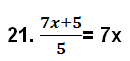Solution:

LHS = (7x+5)/5 = (7x/5)+ 5/5 = (7x/5)+1 ≠ 7x = RHS

The correct statement is (7x+5)/5 = (7x/5) +1

Access Class-8 Chapter-wise Solutions: